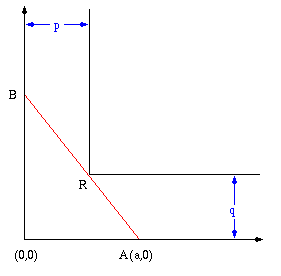# A Tricky Visual Calculus Problem

Calculus Level 2What is the approximate length of the longest ladder (in feet) you can carry horizontally around the corner of the corridor shown here?

$AB$ is the ladder and the coordinate of $R$ is $(8,6)$.

×